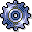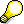##Calculating the Consumption Data for Vehicles### Use

If the costing procedure for vehicles is maintained correctly, you can display consumption data for the vehicle. This section describes what data is determined and how it is calculated. For more information, see Fleet Management.

The system determines the following data:

·        Total counter reading of the primary counter

The system determines the overall counter reading for the primary counter from the measurement document last entered for this counter.

·        Total counter reading of the consumption-relevant fuel counter

The system determines the overall counter reading for the consumption-relevant fuel counter from the measurement document last entered for this counter.

·        Average daily vehicle activity (for example, the distance traveled in kilometers per day) in the long and short term

The calculation of average values for the short and long periods defined in the costing procedure is determined using the first and last measurement documents within this period. For this reason, there must be at least two measurement documents for a counter within the period to give a difference between the start and end value (from which the respective average values are calculated). In addition, both of these documents must have a different date as their measurement times.Note that the measurement value of the first document is always taken as the initial value for showing the difference, but is not, however, itself entered in the calculation of the average values. In Example: Calculation of Consumption Data, the example is concerned with the measurement documents of January 01. If you also want to include this document in the calculation, you must first create an initial document ("zero document", in the case of a new vehicle) as the parent document (for the start date of the productive use of the vehicle), for example:

·         Parent document for the primary counter: 01 January: 0 km

·         Parent document for the fuel counter: 01 January: 0 l

In order to calculate the average daily fleet object activity, the system first determines the start of the period for which the data should be determined.

In the case of a short-term analysis, the start of the period is the current date minus the number of days for the short-term period. You have assigned this number of days to the calculation method in Customizing.

In the case of a long-term analysis, the start of the period is the current date minus the number of days for the long-term period. You have assigned this number of days to the calculation method in Customizing.

The average daily consumption of the vehicle is calculated

¡        From the first recorded measurement document after the beginning of the period (for the primary counter)

¡        And from the last recorded measurement document after the beginning of the period (for the primary counter)

The number of days between the entry dates for these documents is chosen as the time interval.The following formula is used for the calculation:

Average daily activity = (Overall counter reading for the last measurement document – Overall counter reading for the first measurement document after the start of the period) / Number of days between the entry dates for both documents

·        Average daily fuel consumption (for example, liters per day) in the short and long term

In order to calculate the quantity of fuel consumed daily, the system first determines the start of the period for which the data should be determined.

In the case of a short-term analysis, the start of the period is the current date minus the number of days for the short-term period. You have assigned this number of days to the calculation method in Customizing.

In the case of a long-term analysis, the start of the period is the current date minus the number of days for the long-term period. You have assigned this number of days to the calculation method in Customizing.

The average daily fuel consumption is calculated

¡        From the first recorded measurement document after the beginning of the period (for the consumption-relevant fuel counter)

¡        From the last recorded measurement document after the beginning of the period (for the consumption-relevant fuel counter)

The number of days between the entry dates for these documents is chosen as the time interval.The following formula is used for the calculation:

Average daily consumption = (Overall counter reading for the last measurement document – Overall counter reading for the first measurement document after the start of the period) / Number of days between the entry dates for both documents

·        Average vehicle consumption (for example, how many liters on average does the vehicle consume per 62.14 mi? How many liters does the vehicle consume on average per operating hour? How many miles on average can a vehicle travel with 100 liters of fuel?)

In order to calculate the average consumption for the fleet object, the system first determines the start of the period for which the data should be determined.

In the case of a short-term analysis, the start of the period is the current date minus the number of days for the short-term period. You have assigned this number of days to the calculation method in Customizing.

In the case of a long-term analysis, the start of the period is the current date minus the number of days for the long-term period. You have assigned this number of days to the calculation method in Customizing.

Next, the first and last measurement documents recorded after the beginning of the period are determined for the consumption-relevant fuel counter. The difference between the overall counter readings for these measurement documents is displayed.  In addition, the system searches for the measurement document of the primary counter that was recorded closest in time for both of these measurement documents. The difference between the overall counter readings is also displayed in this case. The average consumption is calculated from the quotient of both differences. The unit is expressed in the units that you have assigned to the costing method selected.In order to ensure the precise calculation of these consumption values, you must enter a measurement document for the primary counter close in time for each measurement document entered for the consumption-relevant fuel counter in your system. Ideally, when you refuel the vehicle you should record the kilometer reading, and so on, at the same time.

You can use the original measurement value recording or the consumption-relevant counter-reading recording to record the measurement documents. The latter alternative allows you to perform an integrated (simultaneous) recording of all the measurement documents required for the consumption recording of a vehicle.

·        Next Planned Maintenance Date

The next maintenance date and the number of the maintenance order are only displayed if you set the maintenance planning.

All average values are calculated as an average of a long and a short period, the exact length of which is defined in Customizing. You can use the comparison of long-term and short-term analysis values to detect damages to the vehicle (for example, a leak or a faulty engine) or to recognize misuse.

### Prerequisites

For further information on prerequisites, see Fleet Management and Calculating the Consumption Data for “Miles per Gallon”.